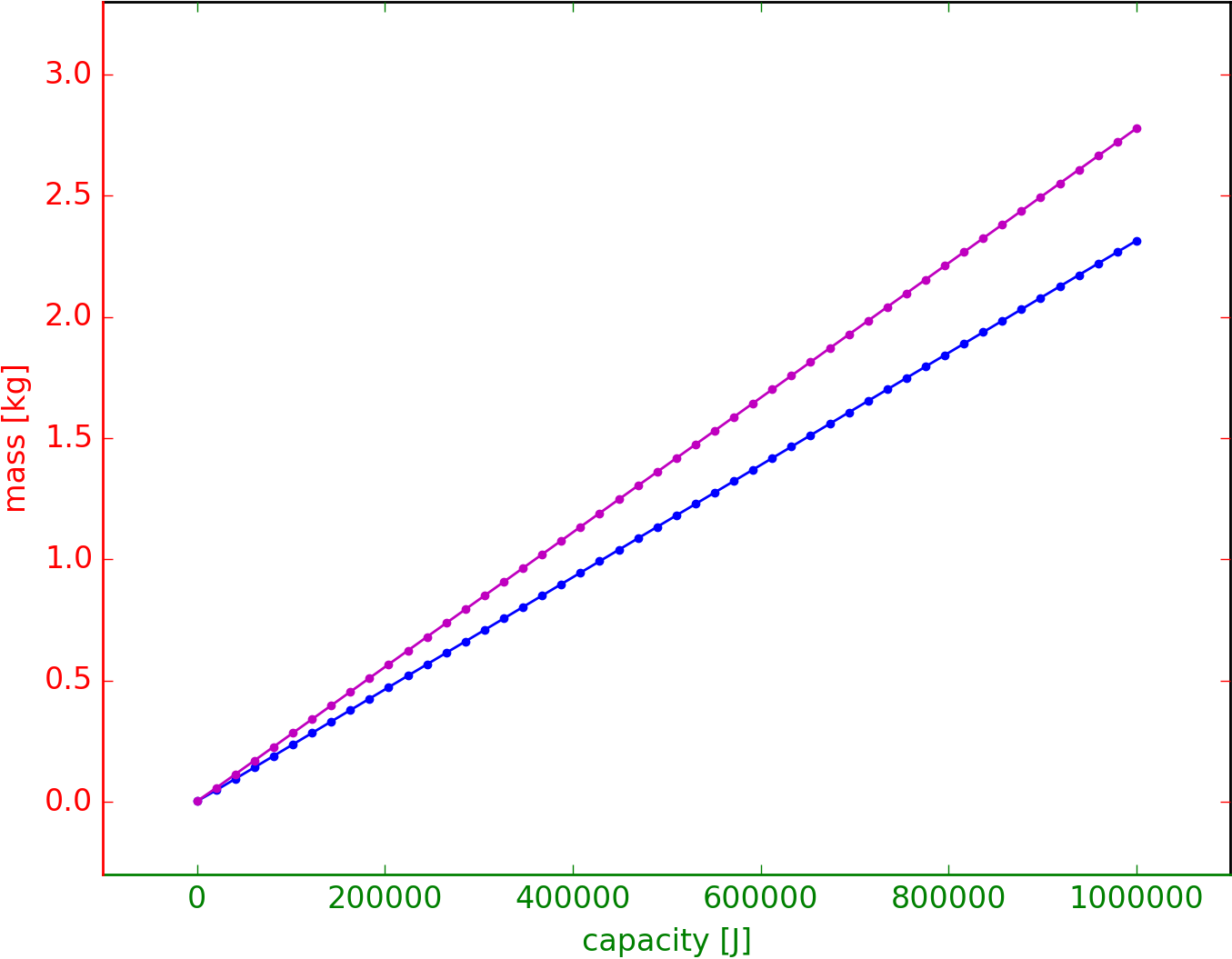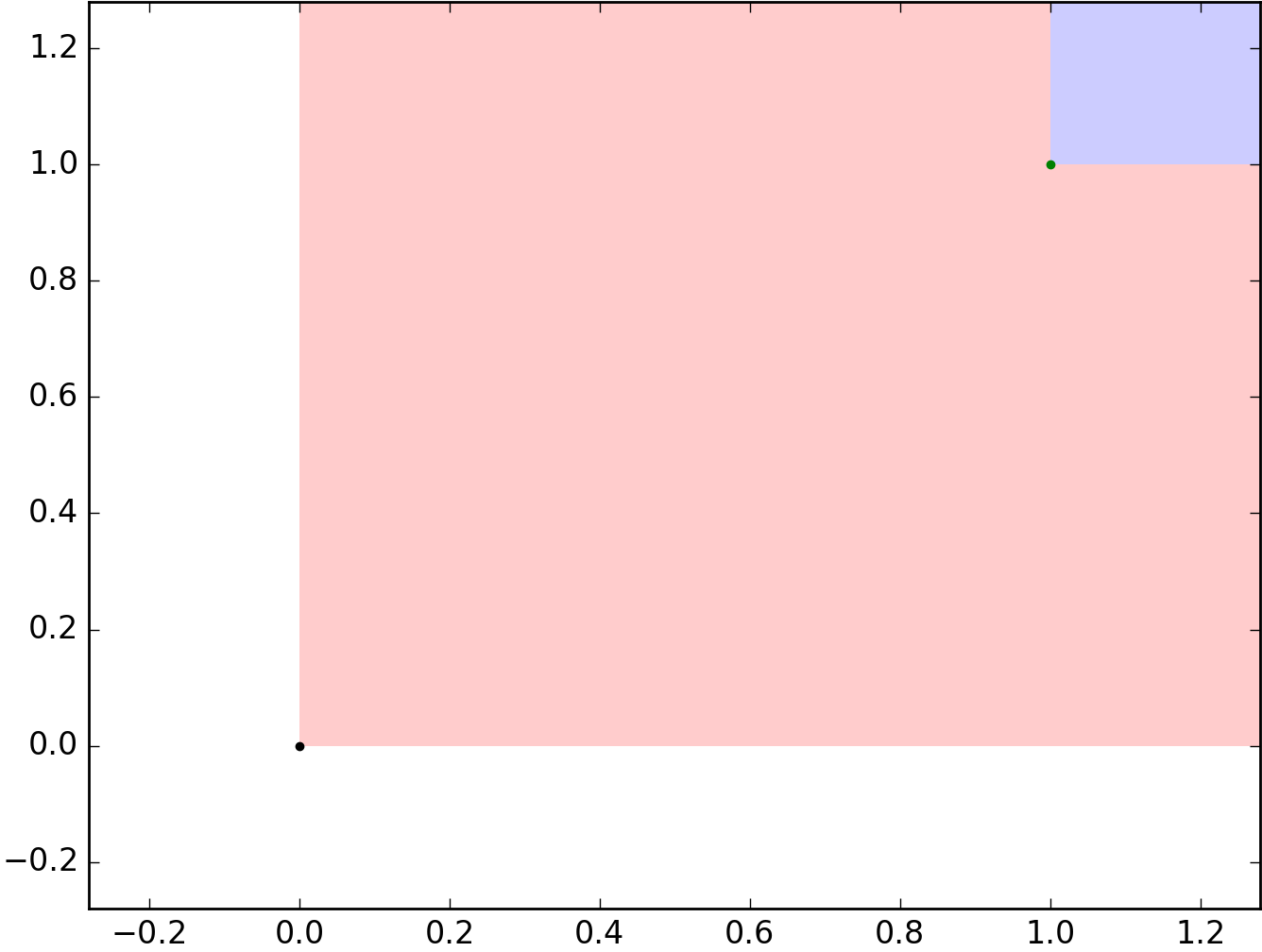# A quick tour

## Describing Monotone Co-Design Problems (MCDPs)

The simplest MCDP can be defined as:

mcdp {

}

That is an empty MCDP - it has no functionality or resources.

The interface of an MCDP is defined using the keywords provides and requires:

mcdp {
provides capacity [J]
requires mass [g]

# ...
}

The code above defines an MCDP with one functionality, capacity, measured in joules, and one resource, mass, measured in grams. (See how to describe types.)

Graphically, this is how the interface is represented:

### Constant functionality and resources

The following is a minimal example of a complete MCDP. We have given hard bounds to both capacity and mass.

 mcdp { provides capacity [J] requires mass [g] provided capacity <= 500 J required mass >= 100g } G cluster1 cluster2 cluster6 node5 capacity [J] node4 500 J node5->node4 node3 100 g node7 mass [g] node3->node7

### Describing relations between functionality and resources

Functionality and resources can depend on each other using any monotone relations.

For example, we can describe a linear relation between mass and capacity, given by the specific energy.

mcdp {
provides capacity [J]
requires mass [g]

specific_energy = 4 J / g
required mass >= provided capacity / specific_energy
}

### Units

PyMCDP is picky about units, but generally very helpful. As long as the units have the right dimensionality, it will insert the appropriate conversions.

For example, this is the same example with the specific energy given in kWh/kg.

mcdp {
provides capacity [J]
requires mass [g]

specific_energy = 200 kWh / kg
required mass >= provided capacity / specific_energy
}

## Composing MCDPs

Suppose we define a simple model called Battery as follows:

 Battery.mcdpmcdp { provides capacity [J] requires mass [g] specific_energy = 100 kWh / kg required mass >= provided capacity / specific_energy } G cluster1 cluster4 node3 capacity [J] node2 Battery node3->node2 node5 mass [g] node2->node5

Let’s also define the MCDP Actuation1:

 Actuation1.mcdpmcdp { provides lift [N] requires power [W] l = lift p0 = 5 W p1 = 6 W/N p2 = 7 W/N^2 required power >= p0 + p1 * l + p2 * l^2 } G cluster1 cluster4 node3 lift [N] node2 Actuation1 node3->node2 node5 power [W] node2->node5

Then we can combine these two together.

We can re-use previously defined MCDPs using the keyword new. This creates two sub-design problems, for now unconnected.

 mcdp { actuation = new Actuation1 battery = new Battery } G cluster1 node2 battery node7 node2->node7 mass [g] node3 actuation node6 node3->node6 power [W] node4 node4->node3 lift [N] node5 node5->node2 capacity [J]

To create a complete MCDP, take “endurance” as a high-level functionality. Then the energy required is equal to endurance × power.

mcdp {
actuation = new Actuation1
battery = new Battery

# battery must provide power for actuation
provides endurance [s]
energy = provided endurance * (power required by actuation)

capacity provided by battery >= energy
}

We can create a model with a loop by introducing another constraint.

Take extra_payload to represent the user payload that we must carry.

Then the lift provided by the actuator must be at least the mass of the battery plus the mass of the payload times gravity:

 Composition.mcdpmcdp { actuation = new Actuation1 battery = new Battery # battery must provide power for actuation provides endurance [s] energy = provided endurance * (power required by actuation) capacity provided by battery >= energy # actuation must carry payload + battery provides payload [g] gravity = 9.81 m/s^2 total_mass = (mass required by battery + provided payload) weight = total_mass * gravity lift provided by actuation >= weight # minimize total mass requires mass [g] required mass >= total_mass } G cluster1 cluster2 cluster19 node17 endurance [s] node7 node17->node7 node18 payload [g] node4 node18->node4 node3 × 9.81000 m/s² node14 node3->node14 [g*m/s²] node16 node4->node16 [g] node5 battery node10 node5->node10 mass [g] node6 actuation node12 node6->node12 power [W] node11 node7->node11 [J] node8 node15 node8->node15 [N] node9 node13 node9->node13 [g] node20 mass [g] node9->node20 node10->node4 [g] node11->node5 capacity [J] node12->node7 [W] node13->node3 [g] node14->node8 [g*m/s²] node15->node6 lift [N] node16->node9 [g]

## Catalogues

We can also enumerate an arbitrary relation, as follows:

catalogue {
provides capacity [J]
requires mass [g]

model1 |  5 MJ | 100 g
model2 |  6 MJ | 200 g
model3 | 10 MJ | 400 g
}

## Coproducts (alternatives)

The coproduct construct allows to describe the idea of “alternatives”. The name comes from the category-theoretical concept of coproduct.

As an example, let us consider how to model the choice between different battery technologies.

Let us consider the model of a battery in which we take the functionality to be the capacity and the resources to be the mass [g] and the cost [$]. Consider two different battery technologies, characterized by their specific energy (Joules per gram) and specific cost (USD per gram). Specifically, consider Nickel-Hidrogen batteries and Lithium-Polymer batteries. On technology is cheaper but leads to heavier batteries and viceversa. Because of this fact, there might be designs in which we prefer either. First we model the two battery technologies separately as two MCDP using the same interface (same resources and same functionality).  Battery_LiPo.mcdpmcdp { provides capacity [J] requires mass [g] requires cost [$] specific_energy = 150 Wh/kg specific_cost = 2.50 Wh/$required mass >= provided capacity / specific_energy required cost >= provided capacity / specific_cost } Battery1_NiH2.mcdpmcdp { provides capacity [J] requires mass [g] requires cost [$] specific_energy = 45 Wh/kg specific_cost = 10.50 Wh/\$ required mass >= provided capacity / specific_energy required cost >= provided capacity / specific_cost } G cluster1 cluster4 node3 capacity [J] node2 Battery1_LiPo node3->node2 node5 cost [USD] node2->node5 node6 mass [g] node2->node6 G cluster1 cluster4 node3 capacity [J] node2 Battery1_NiH2 node3->node2 node5 cost [USD] node2->node5 node6 mass [g] node2->node6

Then we can define the coproduct of the two using the keyword choose. Graphically, the choice is indicated through dashed lines.

 Batteries.mcdpchoose( NiH2: Battery1_LiPo, LiPo: Battery1_NiH2 ) G cluster1 cluster2 cluster9 node8 capacity [J] node5 node8->node5 node3 node11 mass [g] node3->node11 node4 node10 cost [USD] node4->node10 node6 NiH2 node5->node6 node7 LiPo node5->node7 node6->node3 node6->node4 node7->node3 node7->node4

## Describing uncertain MCDPs

The keyword Uncertain is used to define uncertain relations.

For example, suppose there is some uncertain in the value of the specific energy, varying between 100 Wh/kg and 120 Wh/kg. This is one way to describe such uncertainty:

mcdp {
provides capacity [Wh]
requires mass     [kg]

required mass >=
Uncertain(provided capacity/120 Wh/kg ,
provided capacity/100 Wh/kg )

}

The resulting MCDP has an uncertainty gate, marked with “?”, which joins two branches, the optimistic and the pessimistic branch.# Reference

## Describing Posets

All values belong to posets.

PyMCDP knows a few built-in posets, and gives you the possibility of creating your own.

### Natural numbers

The natural numbers with a completion are expressed as Nat and their values using the syntax Nat:42.

### Floating point numbers

Floating point with completion are indicated by R, and their values as 42 [].

Floating point with completion and units are indicated using units, such as:

g, J, m, s, m/s, …

Their values are indicated as follows:

10 g, 20 J, 10 m, 10 s, 23 m/s, …

### Finite Posets

It is possible to define and use your own arbitrary finite posets.

For example, create a file named my_poset.mcdp_poset containing the following definition:

my_poset.mcdp_posetfinite_poset {
a <= b <= c
c <= d
c <= e
}

This defines a poset with 5 elements a, b, c, d, e and with the given order relations.

Now that this poset has been defined, it can be used in the definition of an MCDP, by referring to it by name using the backtick notation, as in “my_poset”.

To refer to its elements, use the notation my_poset: element.

For example:

 mcdp { provides f [my_poset] f <= my_poset : c } G cluster1 cluster2 node4 f [my_poset] node3 'c' node4->node3

### Poset Products

Use the Unicode symbol “×” or the simple letter x to create a poset product.

The syntax is

space × space × … × space


For example:

J × A


This represents a product of Joules and Amperes.

### Tuple making

To create a tuple, use angular brackets.

The syntax is:

< element, element, … >
⟨ element, element, … ⟩


An example using regular brackets:

<0 J, 1 A>

An example using fancy unicode brackets:

⟨0 J, 1 A⟩

### Tuple accessing

To access the elements of the tuple, use the syntax

take(value, index)


For example:

mcdp {
provides out [ J x A ]

take(out, 0) <= 10 J
take(out, 1) <= 2 A
}

This is equivalent to

mcdp {
provides out [ J x A ]

out <= <10 J, 2 A>
}

### Named Poset Products

PyMCDP also supports named products, in which each entry in the tuple is associated to a name. For example, the following declares a product of the two spaces J and A with the two entries named energy and current.

product(energy:J, current:A)

Then it is possible to index those entries using one of these two syntaxes:

take(value, label)
(value).label


For example:

mcdp {
provides out [ product(energy:J, current:A) ]

(out).energy <= 10 J
(out).current <= 2 A
}

## Operations on MCDPs

### Abstraction and flattening

It is easy to create recursive composition in MCDP.

Composition1.mcdpmcdp {
a = instance mcdp {
c = instance mcdp {
provides f [Nat]
requires r [Nat]
provided f + Nat:1 <= required r
}

provides f using c
requires r for   c
}

b = instance mcdp {
d = instance mcdp {
provides f [Nat]
requires r [Nat]
provided f + Nat:1 <= required r
}

provides f using d
requires r for   d
}

r required by a <= f provided by b
requires r for b
provides f using a
}

We can completely abstract an MCDP, using the abstract keyword.

abstract Composition1

And we can also completely flatten it, by erasing the border between subproblems:

flatten Composition1

## Approximations

### Multiplication dual

mcdp  {
provides a [R]
requires b [R]
requires c [R]
a <= b * c
}`
 $$n=1$$ $$n=3$$ $$n=5$$ $$n=10$$ $$n=25$$